Background

The Problem

How Does It Work?

What You Learned

The =apply function we provide is intended to mimic the semantics of Scheme’s standard apply function. In a nutshell, the simplest use of =apply takes as arguments a variadic function, and a list of inputs. It “applies” the list of inputs in order as arguments to the function, and returns a result which is a value defined by the implementer of the variadic function. That is, what it returns varies depending on the function that is passed in as the first argument.

`(apply <variadic-function> <list-of-inputs>) => <result>`

### Background

A variadic function is a function (i.e., a lambda, whether named or supplied inline as a lambda expression) that takes zero or more arguments. =apply is used to call such functions; whereas =eval is used to call functions with a fixed number of arguments.

The simplest example of a variadic function used in the context of Scheme’s apply form is the “+” function. The function takes zero or more numbers, and adds them up. In concept, this is identical to Excel’s handy =SUM function.

```(apply + '(1 3 2 3 2 1)) => 12
(apply + '()) => 0```

To define a variadic function in Scheme, the following form is used:

```(define my-variadic-function
(lambda lst
;; Body of code here, which treats parameters passed in as a list
))```
Note that the parentheses usually placed around the formal arguments of a lambda are missing here around lstThis tells Scheme this lambda is variadic—it can take zero or more inputs.

In the interest of completeness we will merely note here that there is another kind of variadic function definition, that takes some fixed number of initial arguments, and then a variable list of additional/optional arguments. This kind of function definition will look like this in Scheme:

```(define my-variadic-function-with-fixed-and-optional-args
(lambda (arg1 arg2 . rest)
;; Body of code here, which treats arg1 and arg2 as required, and the “rest” as optional
))```

For More Advanced Users: This kind of definition will be helpful when you are writing meta functions of your own that are similar to apply. Most standard Scheme meta functions like apply, map, etc., are in fact implemented in this way, or are macros that produce this kind of function definition. Macros are an advanced subject in Scheme and any proper Lisp, and are arguably every Lisp’s “superpower”; but this topic of metaprogramming with macros (or what Scheme prefers to call “syntax extensions”) will be of interest to developers mainly, and covered elsewhere.

For the purpose of this tutorial, we assume you understand advanced Excel formula writing, maybe even VBA macros, and want to know how =apply works, why only 1-dimensional range references seem to work with it, and what you can do about it with a very small amount of code that’s easy to remember how to write.

### The Problem

In this tutorial, we are focusing on the input dimensionality to the =apply function, and whether or not the ordering of the inputs matters at all. In this case, whether this ordering is expected to be oriented on by-row or by-column semantics is the key issue to be dealt with.

When calling =apply you will tend to provide as the input list either a list literal or a range reference. As long as the list literal or the range references you pass in are “1 dimensional” (meaning, a simple list of heterogeneous inputs), =apply will work just fine.

`=apply("+", {1, 2, 3, 4}) => 10`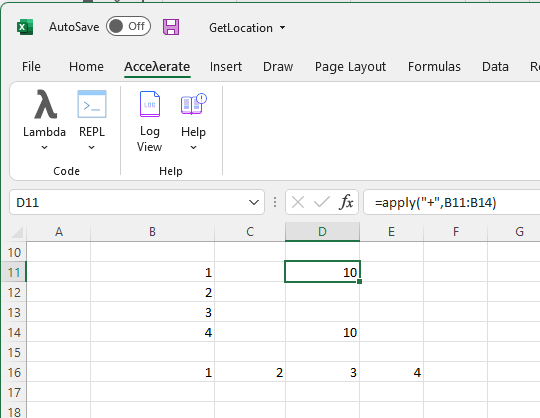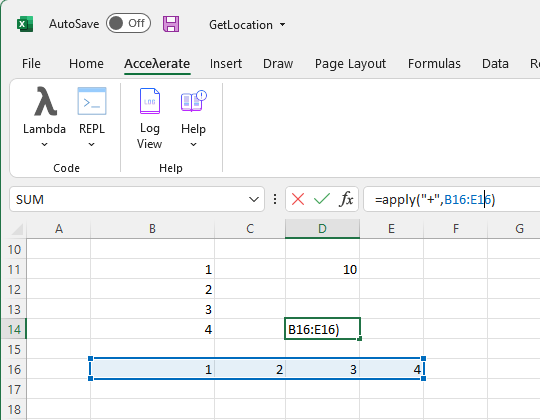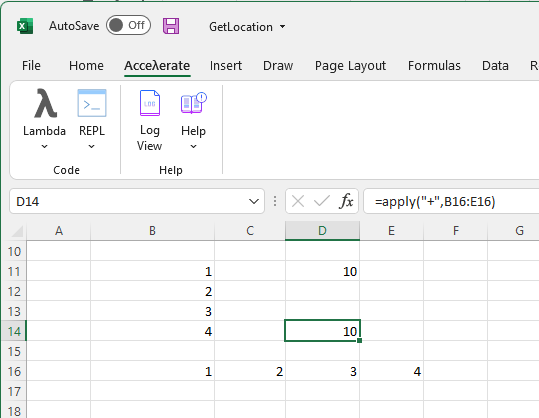However, the minute you pass anything “2-dimensional” like a table range or a “list of lists” list literal, this will not work in the manner in which you hope. The + function will typically return “not a number,” which is true: the input list is in fact a list of lists, not a simple list of number values, which is what expects, when you enter a range spanning more than one row or more than one column, or both.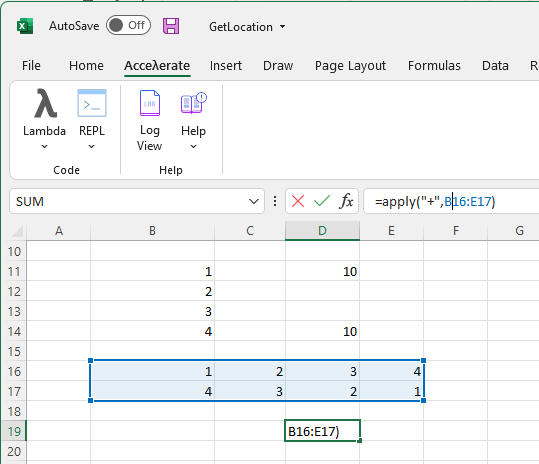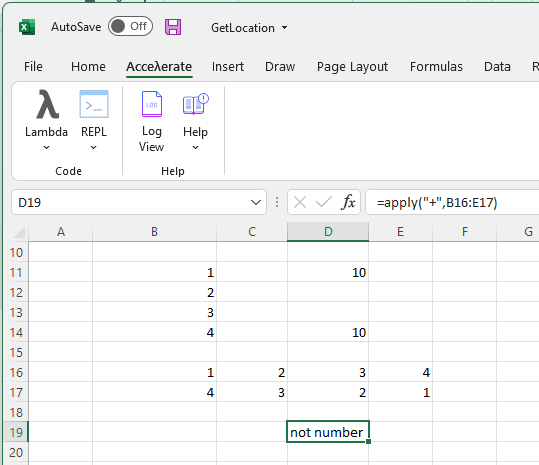What is happening here is that you are passing a 2-dimensional range, and Scheme reads this range in this manner:

`'((1 2 3 4) (4 3 2 1))`

That is, it’s a list of lists. But what “+” expects is:

`'(1 2 3 4 4 3 2 1)`

The Solution

In the case of “+”, order doesn’t really matter. So how do we get this to work? We need to flatten the “list of lists” into a single list of input values, depicted above. Be aware that internally the implicit bias in Excel is to treat 2D regions with “by-row” semantics, which for “+” is good enough. We do not expect a different order of the same inputs to produce anything but 20 as the return value, so let’s focus on dealing with this, as for most situations, this will resolve the issue at hand.

What we need to do is create a lambda function that calls flatten, a utility function that we provide in the visualscheme data helpers library. We automatically load this library on startup for you, so you don’t need to do anything special to access it.

Note: If you use the command line adb.exe, it is not loaded by default; but you can add it to the (import …) spec of your init.ss file in the default working directory of your installation, in which case it will be preloaded in both environments.

Overriding D19, we’ll implement a simple lambda that accepts a list of lists, calls flatten on the input, and uses the flattened version to call apply with the function directly. Since the call to =apply is the last expression, what we get back should be what we want, which is 20.

For the purpose of this example, we are using this as a one-off proof of concept, therefore we will not give it a name and use the  function. In place of the failed call to =apply in D17, press the λ Lambda button in the Acceλerate tab, click Insert Lambda and change the boilerplate to:

```(lambda (lol)
(let ((flattened-lol (flatten lol)))
(apply + flattened-lol)))```

Then update the Lambda Arguments to accept the 2D range, in this case, B16:E17. Then click Accept.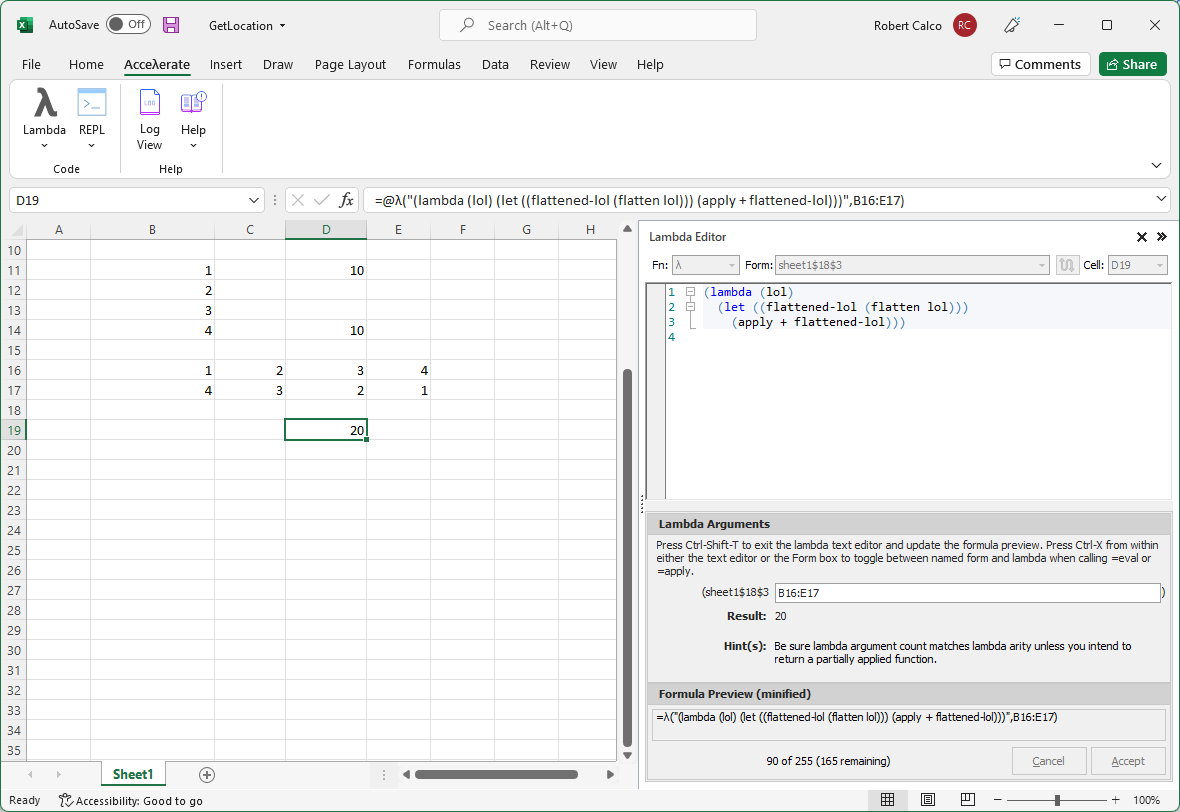### How Does It Work?

The flatten function shares Excel’s row-wise bias and transforms the list of lists into a single list by essentially concatenating the two lists provided in order. Our lambda uses a let binding to get the “flattened” version of the list of lists, and that is provided as input to a call to apply with the function.

But what if order does matter, and we want by-column semantics? Rather than contrive an example, we’ll just demonstrate how to do it. For this, we’ll use the REPL pane. First, make sure the functions are loaded in the environment as they should be. You can do this by typing the expected function names and submitting them with CTRL-SHIFT-T, one at a time. The REPL will show the function signature. Notice they all expect a list of lists, denoted by the ‘lol’ argument.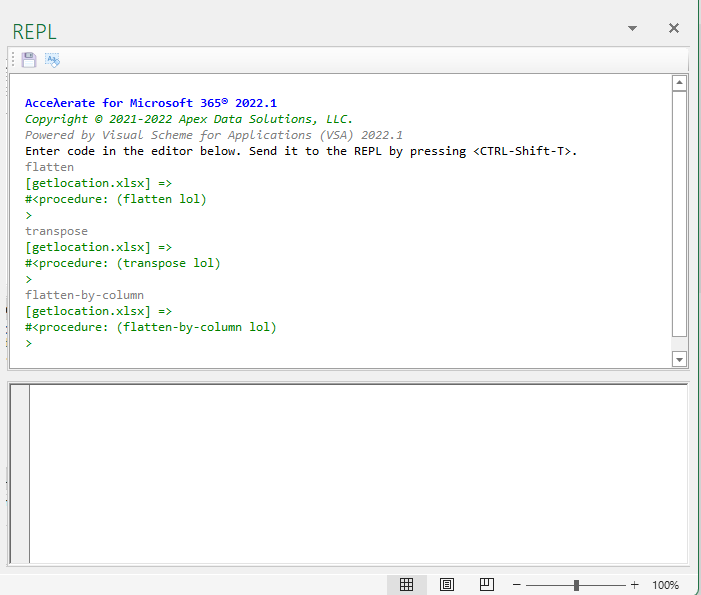The flatten function takes a list of lists and returns a single list. So first let’s define a list of lists:

```(define lol '((1 2 3 4) (4 3 2 1))) => ; the symbol 'lol' is bound to the provided list of lists
lol => ((1 2 3 4) (4 3 2 1))

```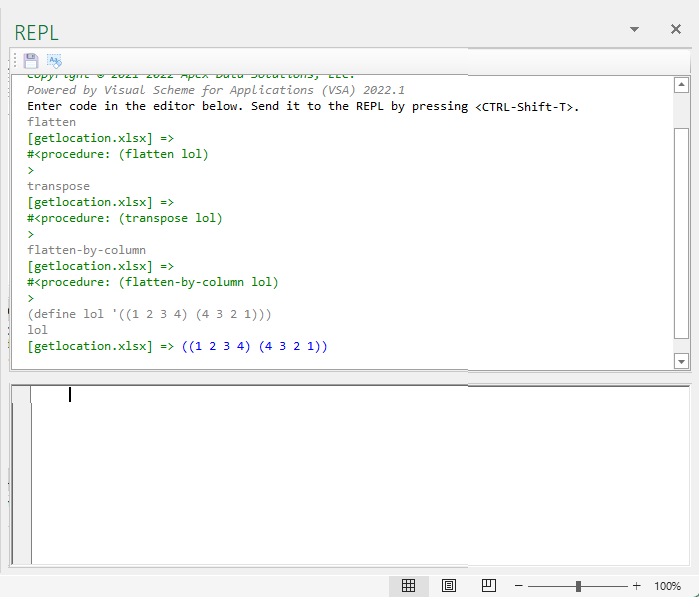Great now let’s call flatten with ‘lol’ to see what it returns:

`(flatten lol) => (1 2 3 4 4 3 2 1)`Now let’s try transpose. The transpose function takes a row-wise list of lists, and turns it into a column-wise list of lists. This is best explained by just seeing it in action:

`(transpose lol) => ((1 4) (2 3) (3 2) (4 1))`The flatten-by-column function uses transpose internally to take create a transposed column-wise list of lists, and return a single flattened list preserving that order:

`(flatten-by-column lol) => (1 4 2 3 3 2 4 1)`

Therefore, to use “by-column” semantics, your lambda would simply call (flatten-by-column lol) on the input range instead of (flatten lol):

```(lambda (lol)
(let ((flattened-lol (flatten-by-column lol)))
(apply + flattened-lol)))```

In this case, the function for this order doesn’t give a different result, but a variadic function for which order is important, and if you want to use column-wise ordering instead of row-wise ordering, you would write your simple lambda that way and it will work.

### What You Learned

To summarize what you have learned with this tutorial:

• You learned about the =apply function, which is based on Scheme’s built in apply function.

• You learned that as long as your input to =apply is a 1-dimensional range (whether by row or by column), =apply will generally work; but if you supply a 2-dimensional range, the function you call with =apply may not like to deal with the resulting “list of lists”.

• You learned that to mitigate this situation, you need to flatten the list and supply the flattened version of it to =apply in the way that you wanted to call =apply originally.

• You learned that the actual code to this is so simple because we implemented the functions you need in the visualscheme data helpers library, and that you can accomplish this by using the =λ function for a quick one-off to get what you want.

The following terms are registered trademarks of the Microsoft group of companies and are used in accordance with Microsoft’s Trade and Brand Guidelines: Microsoft, Microsoft 365, Microsoft Office, Microsoft Excel, Microsoft Edge, Microsoft Edge WebView2, Microsoft Windows, Excel, Office 365